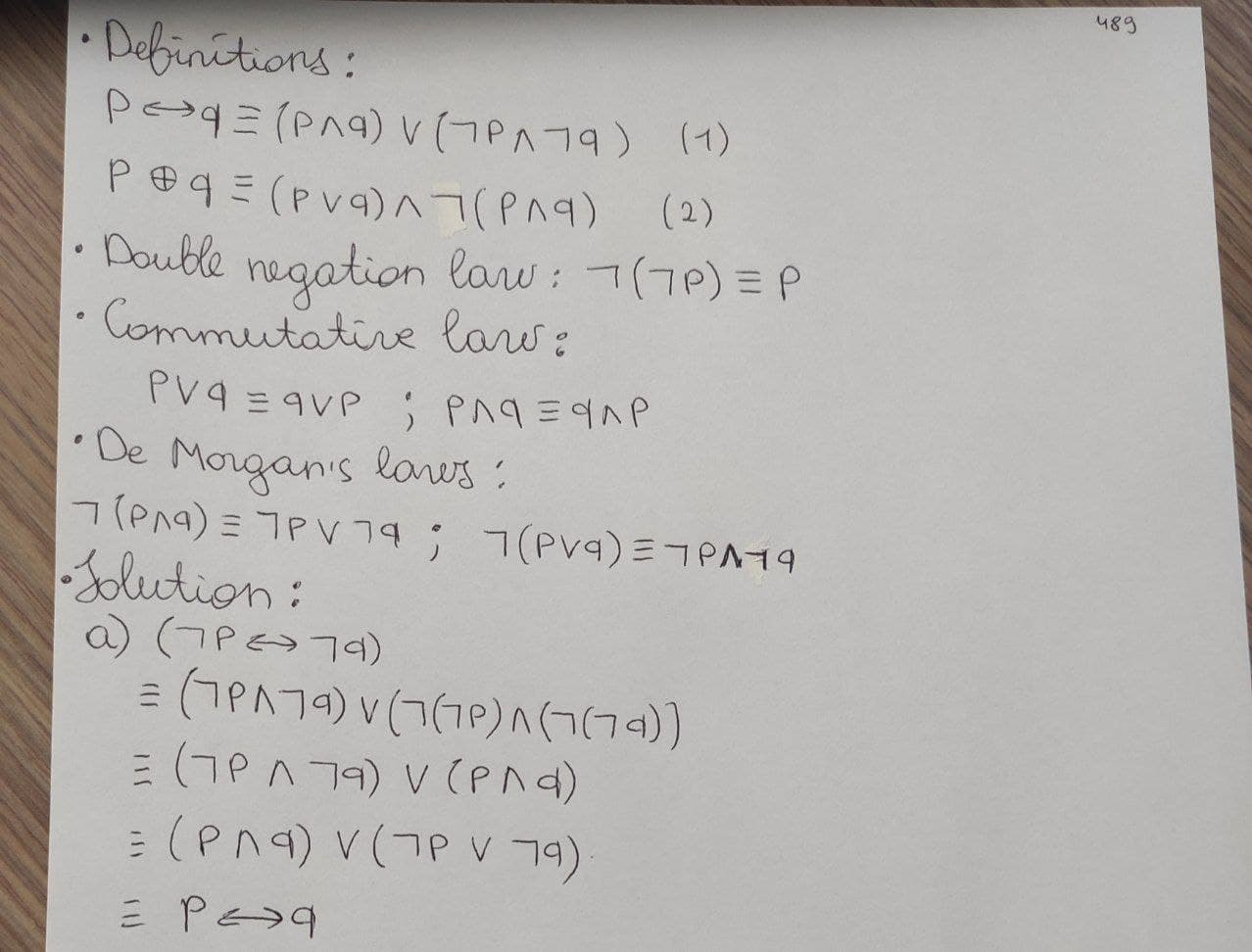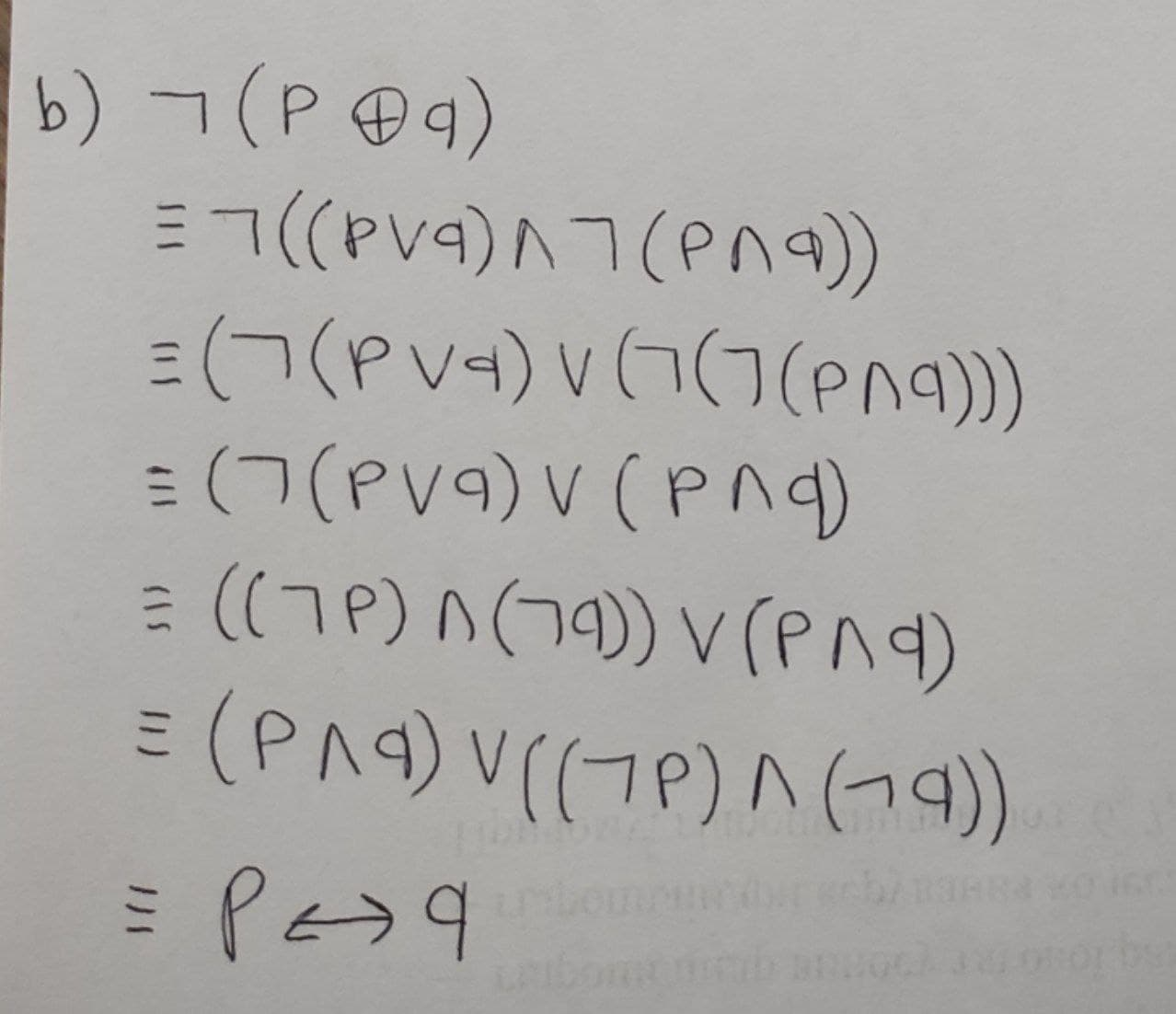# For the following questions you must use the rules of logic (Don’t use truth tables) a) Show that (p harr q) and (not p harr not q ) are logically equivalent. b) Show that not ( p o+ q) and (p harr q) are logically equivalent.permaneceerc 2021-02-19 Answered
For the following questions you must use the rules of logic (Don’t use truth tables)
a) Show that ($p↔q$) and ($\mathrm{¬}p↔\mathrm{¬}q$ ) are logically equivalent.
b) Show that not ( $p\oplus q$) and ($p↔q$) are logically equivalent.
You can still ask an expert for help

## Want to know more about Discrete math?

• Questions are typically answered in as fast as 30 minutes

Solve your problem for the price of one coffee

• Math expert for every subject
• Pay only if we can solve itBrittany Patton
Definitions
$p↔q\equiv \left(p\wedge q\right)\vee \left(\mathrm{¬}p\wedge \mathrm{¬}q\right)$ (1)
$p\oplus \equiv \left(p\vee q\right)\vee \mathrm{¬}\left(p\wedge q\right)$ (2)
Double negation law: $\mathrm{¬}\left(\mathrm{¬}p\right)\equiv p$
Commutative laws: $p\vee q\equiv q\vee p,p\wedge q\equiv q\wedge p$
De Morgan's laws: $\mathrm{¬}\left(p\wedge q\right)\equiv \mathrm{¬}p\vee \mathrm{¬}q,\mathrm{¬}\left(p\vee q\right)\equiv \mathrm{¬}p\wedge \mathrm{¬}q$
a) $\left(\mathrm{¬}p↔\mathrm{¬}q\right)$
$\equiv \left(\mathrm{¬}p\wedge \mathrm{¬}q\right)\vee \left(\mathrm{¬}\left(\mathrm{¬}p\right)\wedge \left(\mathrm{¬}\left(\mathrm{¬}q\right)\right)$
$\equiv \left(\mathrm{¬}p\wedge \mathrm{¬}q\right)\vee \left(p\vee q\right)$
$\equiv \left(p\wedge q\right)\vee \left(\mathrm{¬}p\wedge \mathrm{¬}q\right)$
$\equiv p↔q$
b) $\mathrm{¬}\left(p\oplus q\right)$
$\equiv \mathrm{¬}\left(\left(p\vee q\right)\wedge \mathrm{¬}\left(p\wedge q\right)$
$\equiv \left(\mathrm{¬}\left(p\vee q\right)\right)\vee \left(\mathrm{¬}\left(\mathrm{¬}\left(p\wedge q\right)\right)\right)$
$\equiv \left(\mathrm{¬}\left(p\vee q\right)\right)v\left(p\wedge q\right)$
$\equiv \left(\left(\mathrm{¬}p\right)\wedge \left(\mathrm{¬}q\right)\right)\vee \left(p\wedge q\right)$
$\equiv \left(p\wedge q\right)\vee \left(\left(\mathrm{¬}p\wedge \left(\mathrm{¬}q\right)\right)$
$\equiv p↔q$